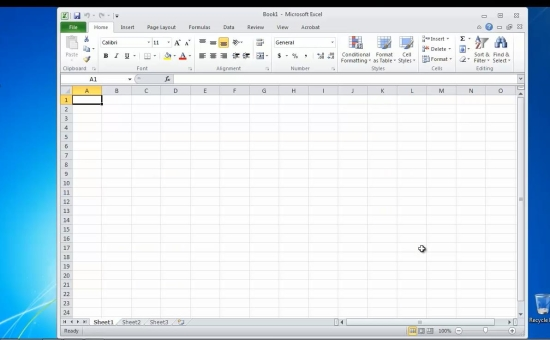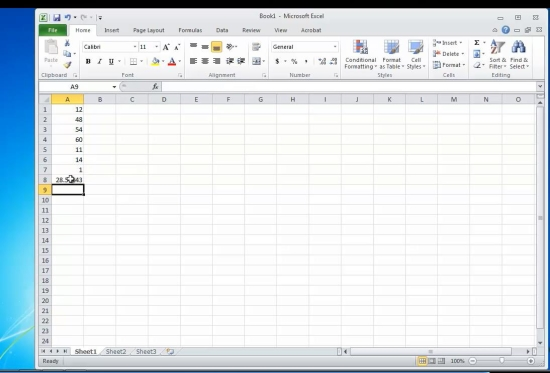## How to Calculate the Mean in Excel

In this tutorial you will learn how to calculate the mean in Excel.

Calculating the mean from a set of numbers is done by the formula:

Mean (number set) = Sum (components)/number of elements, or

This means you need a set of numbers to calculate the means for.

Prerequisites: Microsoft Excel.

Step 1. Let’s create it:

Launch Excel and start a new file from File- New.Step 2. Fill several rows with the various values you want to calculate the Mean for.Step 3. At the ending row, add the following formula:

= =SUM(your data set)/COUNT(your data set), where your data set is the number set you have selected.

Hit Enter. The Mean of your input numbers is displayed.Result: Congratulations, you have learnt how to calculate the mean in Excel.